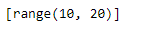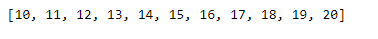Related Articles
range() to a list in Python
• Last Updated : 30 Jan, 2019

Often times we want to create a list containing a continuous value like, in a range of 100-200. Let’s discuss how to create a list using the `range()` function.

Will this work ?

 `# Create a list in a range of 10-20``My_list ``=` `[``range``(``10``, ``20``, ``1``)]`` ` `# Print the list``print``(My_list)`

Output :As we can see in the output, the result is not exactly what we were expecting because Python does not unpack the result of the range() function.

Code #1: We can use argument-unpacking operator i.e. *.

 `# Create a list in a range of 10-20``My_list ``=` `[``*``range``(``10``, ``21``, ``1``)]`` ` `# Print the list``print``(My_list)`

Output :As we can see in the output, the argument-unpacking operator has successfully unpacked the result of the range function.

Code #2 : We can use the `extend()` function to unpack the result of range function.

 `# Create an empty list``My_list ``=` `[]`` ` `# Value to begin and end with``start, end ``=` `10``, ``20`` ` `# Check if start value is smaller than end value``if` `start < end:``    ``# unpack the result``    ``My_list.extend(``range``(start, end))``    ``# Append the last value``    ``My_list.append(end)`` ` `# Print the list``print``(My_list)`

Output :Attention geek! Strengthen your foundations with the Python Programming Foundation Course and learn the basics.

To begin with, your interview preparations Enhance your Data Structures concepts with the Python DS Course.

My Personal Notes arrow_drop_up